Next:9.9 Radix SortingUp:9.8 Lower Bound on Complexity for Sorting MethodsPrevious:9.8.1 Result 1: Lower Bound on Worst Case Complexity

## 9.8.2 Result 2: Lower Bound on Average Case Complexity

We shall show that in any decision tree with K leaves, the average depth of a leaf is at least log K

We shall show the result for any binary tree with K leaves.

Suppose the result is not true. Suppose T is the counterexample with the fewest nodes.

T cannot be a single node because log 1 = 0. Let T have k leaves. T can only be of the following two forms. Now see Figure 9.6.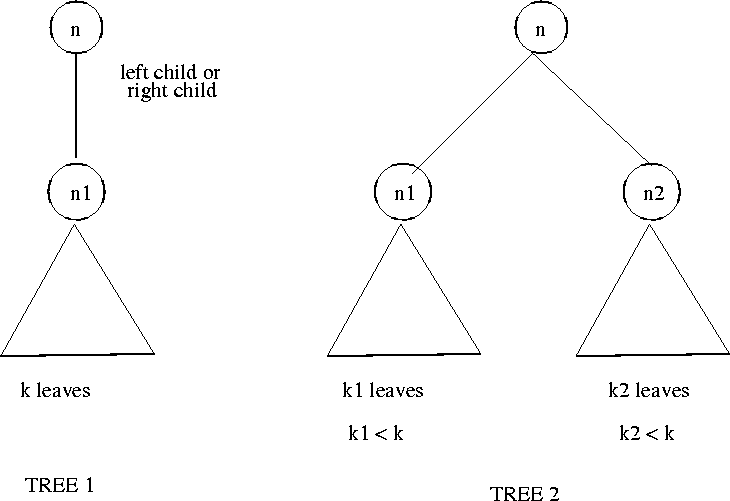Suppose T is of the from Tree 1. The tree rooted at n1, has fewer nodes than T but the same number of leaves and the hence an even smaller counterexample than T. Thus T cannot be of Tree 1 form.

Suppose T is of the form of Trees 2. The trees T1 and T2 rooted at n1 and n2 are smaller than T and therefore the

 Average depth of  T1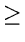logk1 Average depth of  T2logk2

Thus the average depth of T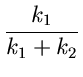logk1 +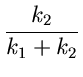logk2 + 1 =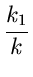logk1 +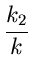logk2 +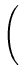+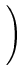=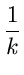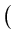k1log 2k1 + k2log 2k2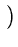logk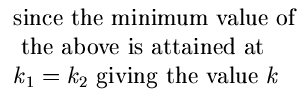This contradicts the premise that the average depth of T is < log k.

Thus T cannot be of the form of Tree 2.

Thus in any decision tree with n! leaves, the average path length to a leaf is at least

log(n!)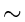O(nlog n)Next:9.9 Radix SortingUp:9.8 Lower Bound on Complexity for Sorting MethodsPrevious:9.8.1 Result 1: Lower Bound on Worst Case Complexity
eEL,CSA_Dept,IISc,Bangalore